# 基于SDN的网络流量异常检测技术Anomaly Detection Technology of Network Traffic in SDN

• 全文下载: PDF(986KB)    PP.1696-1705   DOI: 10.12677/CSA.2018.811187
• 下载量: 485  浏览量: 1,087   国家自然科学基金支持

Software-Defined Networking (SDN) is a novel network architecture that has been successfully developed commercially. However, due to the increasing number and variety of network flows in the information society, abnormal detection of network traffic is becoming important. To realize anomaly detection of traffic in SDN network environment, this paper presents an algorithm based on Support Vector Regression (SVR) and Auto-Regressive Integrated Moving Average (ARIMA). The algorithm makes full use of the characteristics of SDN network, obtains the running state of the network periodically, and uses the ARIMA model to predict, then corrects the prediction results through the SVR model. The experimental results show that ARIMA-SVR model has higher accuracy and detection rate than ARIMA model; and compared with Support Vector Machine (SVM) model, ARIMA-SVR model can detect unknown types of abnormal traffic quickly.

1. 引言

2. 相关工作

3. 模型建立

3.1. 自回归滑动平均模型

${y}_{t}=\underset{k=1}{\overset{p}{\sum }}{\phi }_{k}{y}_{t-k}+{e}_{t}+\underset{k=1}{\overset{q}{\sum }}{\theta }_{k}{e}_{t-k}$ , (1)

ARIMA模型通过对过去时刻的时间序列进行平稳性检查，通过d次差分，将非平稳序列转化为平稳序列，之后使用自相关函数和偏自相关函数确定模型的p、q值范围，再使用BIC最小化原则选择最优的p、q值，最后通过多项式拟合等曲线拟合方法来求得待求系数 ${\phi }_{t}$ 的值。

3.2. 支持向量回归

SVR的核心思想与SVM相同，都是将样本空间通过核函数映射到特征空间中，在特征空间实现对样本的回归。对于数据集 $D=\left\{\left({x}_{1},{y}_{1}\right),\cdots ,\left({x}_{m},{y}_{m}\right)\right\}$ ，其中 ${x}_{i}\in {R}^{n},{y}_{i}\in R,i=1,\cdots ,m$ 。引入松弛变量 $\xi ,{\xi }^{*}$ 、不敏感系数 $\epsilon$ 以及惩罚参数 $C>0$ 。当满足条件 $-\epsilon -{\xi }_{i}^{\ast }\le {y}_{i}-w\cdot {x}_{i}-b\le \epsilon +{\xi }_{i}$ 时，可认为这些数据点都有同样的回归方程。其中w和b为待求系数，则该参数可由如下公式求出：

$\begin{array}{l}\underset{w,b,{\xi }_{i},{\xi }_{i}^{\ast }}{\mathrm{min}}\frac{1}{2}{‖w‖}^{2}+C\underset{i=1}{\overset{m}{\sum }}\left({\xi }_{i}+{\xi }_{i}^{\ast }\right),\\ \text{s}\text{.t}\text{.}\text{\hspace{0.17em}}\left\{\begin{array}{l}{y}_{i}-w\cdot {x}_{i}-b\le \epsilon +{\xi }_{i},\\ w\cdot {x}_{i}+b-{y}_{i}\le \epsilon +{\xi }_{i}^{\ast },\\ {\xi }_{i},{\xi }_{i}^{\ast }\ge 0.\end{array}\end{array}$ (2)

3.3. 异常检测模型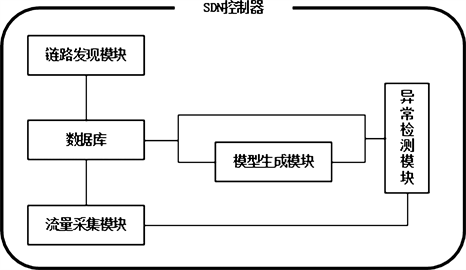Figure 1. Diagram of module in SDN controller

$\text{BandWidth}=\frac{\text{current_byte}-\text{pre_byte}}{1024\ast \text{sampling_time}}$ , (3)

${F}_{t}={y}_{\text{ARIMA}}+{Q}_{\text{SVR}}$ . (4)

1) 控制器使用LLDP协议发现所有链接的交换机。

2) 控制器周期性发送OFPT_PORT_STATUS数据包，获得当前时刻通过交换机各个端口的数据包总字节数。之后利用公式(3)计算已用带宽，并将其保存到数据库中。

3) 选取一定数量的带宽值，使用ARIMA算法训练模型，得到预测值 ${y}_{\text{ARIMA}}$

4) 使用预测值 ${y}_{\text{ARIMA}}$ ，是否周末(W)，是否节日(F)，是否有热点事件(H)这四个变量作为输入特征，通过SVR训练模型并进行预测，得到修正值 ${Q}_{\text{SVR}}$

5) 根据公式(4)得到最终的预测值  。如果下一时刻的真实值，没有超过1.2倍的 ${F}_{t}$ ，则说明当前是正常流量，反之，则说明当前流量有异常。

4. 实验结果与分析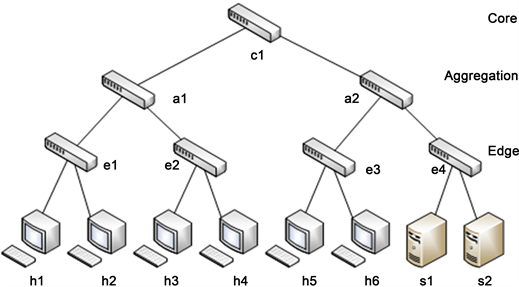Figure 2. Simulation network topology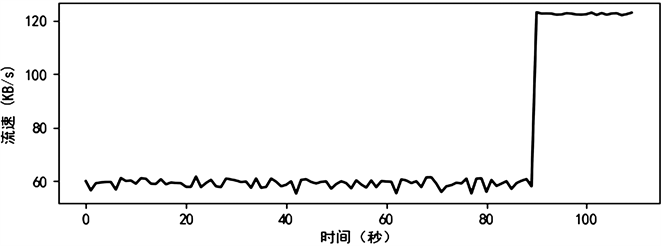Figure 3. Raw network traffic dataTable 1. Stationarity and white noise testTable 2. Related parameters of ARIMA model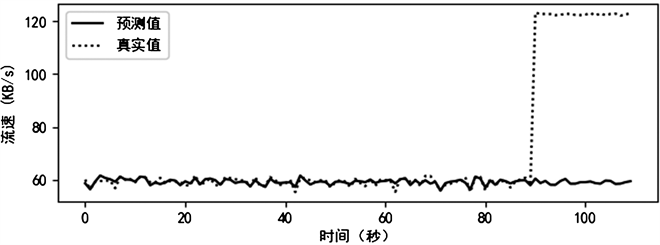Figure 4. Prediction results of ARIMA model

$准确率=\frac{正确匹配的流数}{总的流数}$ . (5)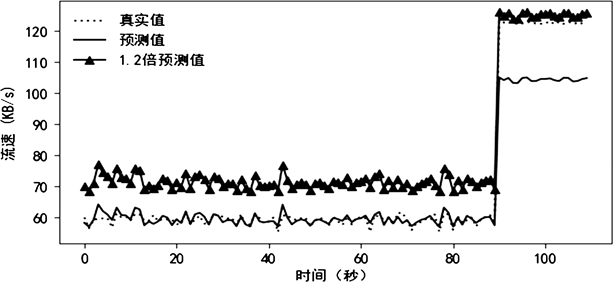Figure 5. Prediction results of ARIMA-SVR model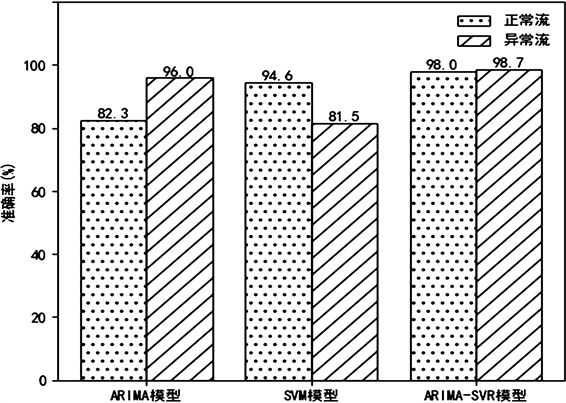Figure 6. Accuracy of each anomaly detection model

$\text{DR}=\frac{异常流中正确分类的流数}{已知的异常流数}$ , (6)

$\text{FAR}=\frac{正常流中错误分类的流数}{所有为果为异常流数}$ . (7)Table 3. Stability of each anomaly detection model

5. 结论

NOTES

*通讯作者。

  Kreutz, D., Ramos, F.M.V., Esteves, V.P., et al. (2014) Software-Defined Networking: A Comprehensive Survey. Proceedings of the IEEE, 103, 10-13.  Liu, C., Hoi, S.C.H., Zhao, P., et al. (2016) Online ARIMA Algorithms for Time Series Prediction. 30th AAAI Conference on Artificial Intelligence, AAAI Press, 1867-1873.  Qian, Y.K. and Chen, M. (2011) MOADA-SVR: A Multivariate Online Anomaly Detection Algorithm Based on SVR. Journal on Communications, 32, 106-113.  Box, G.E.P., Jenkins, G.M., Reinsel, G.C., et al. (2015) Time Series Analysis: Forecasting and Control. 5th Edition, John Wiley and Sons Inc., Hoboken, pp. 712.  杨连群, 宋津旭, 李翔宇. 网络日志和流量关联分析的必要性[J]. 电子技术与软件工程, 2017(14): 11.  王珣. 基于Netflow的局域网流量异常检测系统的设计与实现[J]. 信息与电脑(理论版), 2016(21): 186-188.  曾建华. 一种基于核PCA的网络流量异常检测算法[J]. 计算机应用与软件, 2018, 35(3): 140-144.  王强. SDN网络路由算法及流量监控方法的研究与应用[D]: [硕士学位论文]. 大连: 大连海事大学, 2016.  Wang C., Mei W., Qin X., et al. (2017) Quantum Entropy Based Tabu Search Algorithm for Energy Saving in SDWN. Science China (Information Sciences), 60, 040307. https://doi.org/10.1007/s11432-017-9044-x  王文涛, 王玲霞, 黄烨. SDN环境下基于Renyi熵的低速率分布式拒绝攻击的检测[J]. 中南民族大学学报(自然科学版), 2017, 36(3): 131-136.  Carvalho, L.F., Fernandes, G., Rodrigues, J.J.P.C., et al. (2017) A Novel Anomaly Detection System to Assist Network Management in SDN Environment. IEEE International Conference on Communications, Paris, 21-25 May 2017, 1-6. https://doi.org/10.1109/ICC.2017.7997214  Silva, A.S.D., Wickboldt, J.A., Granville, L.Z., et al. (2016) ATLANTIC: A Framework for Anomaly Traffic Detection, Classification, and Mitigation in SDN. IEEE/IFIP Network Operations and Management Symposium, Istanbul, 25-29 April 2016, 27-35.  Boero, L., Marchese, M. and Zappatore, S. (2017) Support Vector Machine Meets Software Defined Networking in IDS Domain. 29th International Teletraffic Congress (ITC 29), Genoa, 4-8 September 2017, 25-30. https://doi.org/10.23919/ITC.2017.8065806  王晓瑞, 庄雷, 胡颖, 等. SDN环境下基于BP神经网络的DDoS攻击检测方法[J]. 计算机应用研究, 2018, 35(3).  王伟. 基于深度学习的网络流量分类及异常检测方法研究[D]: [博士学位论文]. 北京: 中国科学技术大学, 2018.  徐毅, 曾文兵. Openstack虚拟化流量平台监控系统[J]. 计算机系统应用, 2018(2).  Le, L., Sinh, D., Lin, B.P., et al. (2018) Applying Big Data, Machine Learning, and SDN/NFV to 5G Traffic Clustering, Forecasting, and Management. 4th IEEE Conference on Network Softwarization and Workshops (NetSoft), IEEE, 168-176.  Kataoka, K., Gangwar, S. and Podili, P. (2018) Trust List: Internet-Wide and Distributed IoT Traffic Management Using Blockchain and SDN. IEEE 4th World Forum on Internet of Things (WF-IoT), Singapore, 5-8 February 2018, 296-301. https://doi.org/10.1109/WF-IoT.2018.8355139  Monshizadeh, M., Khatri, V. and Kantola, R. (2017) An Adaptive Detection and Prevention Architecture for Unsafe Traffic in SDN Enabled Mobile Networks. IFIP/IEEE Symposium on Integrated Network and Service Management (IM), Lisbon, 8-12 May 2017.  Nguyen, T.H. and Yoo, M. (2017) Analysis of Link Discovery Service At-tacks in SDN Controller. International Conference on Information Networking, Da Nang, 11-13 January 2017.